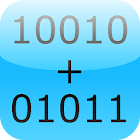Binary Calculator

All Android applications categories

All Android games categories# Binary Calculator

by: 2.5K 8

8 Users
rating

## Screenshots

Description

This is a free mathematical calculator, which is able to add, subtract, multiply and divide numbers in binary format (used in computers).

Any number can be represented by any sequence of bits (binary digits), they are commonly written using the symbols 0 and 1.

Best math tool for school and college! If you are a student, it will helps you to learn arithmetics for computer science and electrical engineering.

Tags: calculator add subtract multiply and divide , binary calculator , бинарный калькулятор , двоичный калькулятор андроид , free binary calculator , calculatrice binaire , convert numbers to binary code , calcolatrice divider radice , binary code calculator

## Users review

from 2.537 reviews

"Great"

8